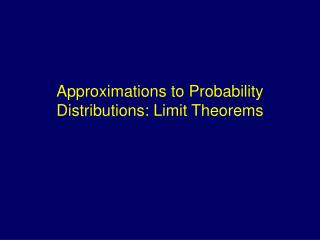DownloadDownload PresentationApproximations to Probability Distributions: Limit Theorems

# Approximations to Probability Distributions: Limit Theorems

Télécharger la présentation## Approximations to Probability Distributions: Limit Theorems

- - - - - - - - - - - - - - - - - - - - - - - - - - - E N D - - - - - - - - - - - - - - - - - - - - - - - - - - -
##### Presentation Transcript

1. Approximations to Probability Distributions: Limit Theorems

2. Sequences of Random Variables • Interested in behavior of functions of random variables such as means, variances, proportions • For large samples, exact distributions can be difficult/impossible to obtain • Limit Theorems can be used to obtain properties of estimators as the sample sizes tend to infinity • Convergence in Probability – Limit of an estimator • Convergence in Distribution – Limit of a CDF • Central Limit Theorem – Large Sample Distribution of the Sample Mean of a Random Sample

3. Convergence in Probability • The sequence of random variables, X1,…,Xn, is said to converge in probability to the constant c, if for every e>0, • Weak Law of Large Numbers (WLLN): Let X1,…,Xn be iid random variables with E(Xi)=m and V(Xi)=s2 < . Then the sample mean converges in probability to m:

4. Proof of WLLN

5. Other Case/Rules • Binomial Sample Proportions • Useful Generalizations:

6. Convergence in Distribution • Let Yn be a random variable with CDF Fn(y). • Let Y be a random variable with CDF F(y). • If the limit as n of Fn(y) equals F(y) for every point y where F(y) is continuous, then we say that Ynconverges in distribution to Y • F(y) is called the limiting distribution function of Yn • If Mn(t)=E(etYn) converges to M(t)=E(etY), then Yn converges in distribution to Y

7. Example – Binomial  Poisson • Xn~Binomial(n,p) Let l=np  p=l/n • Mn(t) = (pet + (1-p))n = (1+p(et-1))n = (1+l(et-1)/n)n • Aside: limn (1+a/n)n = ea •  limn Mn(t) = limn (1+l(et-1)/n)n = exp(l(et-1)) • exp(l(et-1)) ≡ MGF of Poisson(l) •  Xn converges in distribution to Poisson(l=np)

8. Example – Scaled Poisson  N(0,1)

9. Central Limit Theorem • Let X1,X2,…,Xn be a sequence of independently and identically distributed random variables with finite mean m, and finite variance s2. Then: • Thus the limiting distribution of the sample mean is a normal distribution, regardless of the distribution of the individual measurements

10. Proof of Central Limit Theorem (I) • Additional Assumptions for this Proof: • The moment-generating function of X, MX(t), exists in a neighborhood of 0 (for all |t|<h, h>0). • The third derivative of the MGF is bounded in a neighborhood of 0 (M(3)(t) ≤ B< for all |t|<h, h>0). • Elements of Proof • Work with Yi=(Xi-m)/s • Use Taylor’s Theorem (Lagrange Form) • Calculus Result: limn[1+(an/n)]n = ea if limnan=a

11. Proof of CLT (II)

12. Proof of CLT (III)

13. Proof of CLT (IV)

14. Proof of CLT (V)